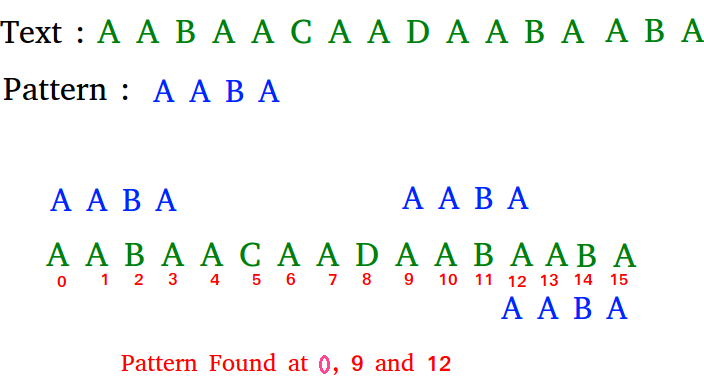# Python Program for KMP Algorithm for Pattern Searching

Given a text txt[0..n-1] and a pattern pat[0..m-1], write a function search(char pat[], char txt[]) that prints all occurrences of pat[] in txt[]. You may assume that n > m
Examples:

```Input:  txt[] = "THIS IS A TEST TEXT"
pat[] = "TEST"
Output: Pattern found at index 10

pat[] =  "AABA"
Output: Pattern found at index 0
Pattern found at index 9
Pattern found at index 12```` `

Pattern searching is an important problem in computer science. When we do search for a string in notepad/word file or browser or database, pattern searching algorithms are used to show the search results.

## Python3

 `# Python program for KMP Algorithm` `def` `KMPSearch(pat, txt):` `    ``M ``=` `len``(pat)` `    ``N ``=` `len``(txt)`   `    ``# create lps[] that will hold the longest prefix suffix ` `    ``# values for pattern` `    ``lps ``=` `[``0``]``*``M` `    ``j ``=` `0` `# index for pat[]`   `    ``# Preprocess the pattern (calculate lps[] array)` `    ``computeLPSArray(pat, M, lps)`   `    ``i ``=` `0` `# index for txt[]` `    ``while` `i < N:` `        ``if` `pat[j] ``=``=` `txt[i]:` `            ``i ``+``=` `1` `            ``j ``+``=` `1`   `        ``if` `j ``=``=` `M:` `            ``print` `(``"Found pattern at index"``, ``str``(i``-``j))` `            ``j ``=` `lps[j``-``1``]`   `        ``# mismatch after j matches` `        ``elif` `i < N ``and` `pat[j] !``=` `txt[i]:` `            ``# Do not match lps[0..lps[j-1]] characters,` `            ``# they will match anyway` `            ``if` `j !``=` `0``:` `                ``j ``=` `lps[j``-``1``]` `            ``else``:` `                ``i ``+``=` `1`   `def` `computeLPSArray(pat, M, lps):` `    ``len` `=` `0` `# length of the previous longest prefix suffix`   `    ``lps[``0``] ``# lps is always 0` `    ``i ``=` `1`   `    ``# the loop calculates lps[i] for i = 1 to M-1` `    ``while` `i < M:` `        ``if` `pat[i]``=``=` `pat[``len``]:` `            ``len` `+``=` `1` `            ``lps[i] ``=` `len` `            ``i ``+``=` `1` `        ``else``:` `            ``# This is tricky. Consider the example.` `            ``# AAACAAAA and i = 7. The idea is similar ` `            ``# to search step.` `            ``if` `len` `!``=` `0``:` `                ``len` `=` `lps[``len``-``1``]`   `                ``# Also, note that we do not increment i here` `            ``else``:` `                ``lps[i] ``=` `0` `                ``i ``+``=` `1`   `txt ``=` `"ABABDABACDABABCABAB"` `pat ``=` `"ABABCABAB"` `KMPSearch(pat, txt)`   `# This code is contributed by Bhavya Jain`

Output:

`Found pattern at index 10`

Time Complexity: O(m+n)

Space Complexity: O(m)

Please refer complete article on KMP Algorithm for Pattern Searching for more details!

Whether you're preparing for your first job interview or aiming to upskill in this ever-evolving tech landscape, GeeksforGeeks Courses are your key to success. We provide top-quality content at affordable prices, all geared towards accelerating your growth in a time-bound manner. Join the millions we've already empowered, and we're here to do the same for you. Don't miss out - check it out now!